Ten Plus Bonds: Building With Three Parts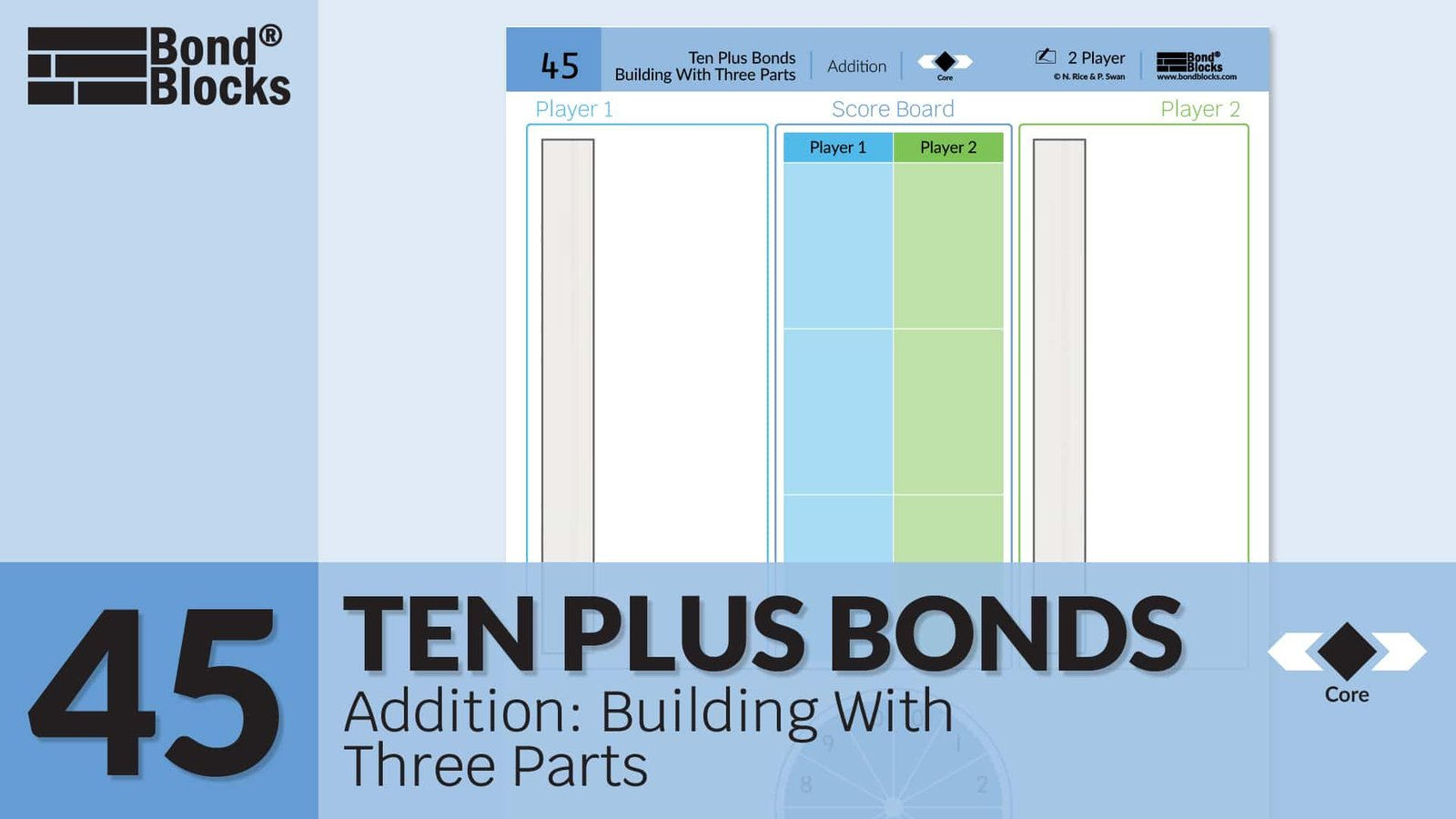### Mathematics

Develop the concept of the associative property of addition for wholes with three parts: parts can be rearranged and added in any order without changing the size of the whole.

• Parts should be rearranged to make addition easier.
• In this activity students rearrange parts to first make a bond of ten. This then creates a ten plus bond.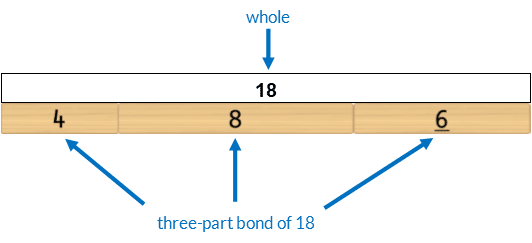The parts of 4, 8 and 6  are rearranged to make addition easier.Develop fluency using bonds of ten and ten plus bonds to add three-part wholes.

### Language

• “equals”
• bond of ten
• ten plus bond
• three-part bond
• partition
• rearrrange
• build

### Core Activity

Blocks in this activity are positioned vertically as per the previous two activities because:

• this activity is an extension of the previous two.
• the vertical positioning supports students to make connections about place value between the quantity (Bond Blocks), written number and value of each digit within the number.

In this activity the student flicks the spinner twice. Each time they collect a matching block.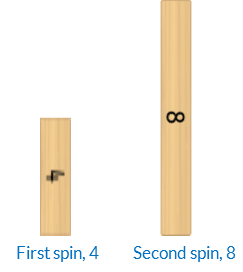The student can use either of these blocks to select a third block. The third block must join with one of the other blocks to build 10. Allow students to make this choice. The blocks must then be positioned to support place value with the bond of 10 on the left and the single block representing the ones value on the right.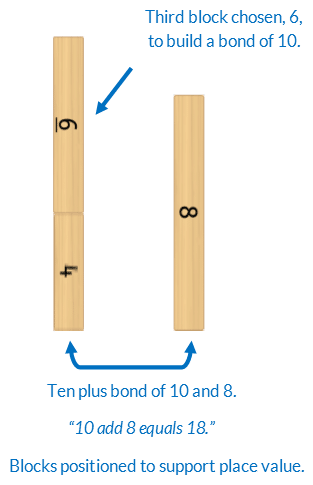It may take students a few rounds to make the best choice to maximise the size of the whole when building ten. For example, instead of choosing the 4 to build ten a player could chose 8. This is also correct but will not result in the largest possible whole.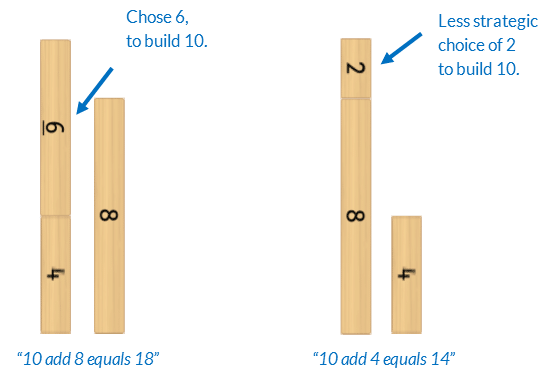Once students have developed fluency playing the game ask them to share and explain strategy choices.## Differentiation

### A little easier

##### Place value arrow cards

Click to download Place Value Arrow CardsUse these with Bond Blocks to support the calculation. Ensure blocks are rearranged to support place value with the tens value on the left and ones value on the right.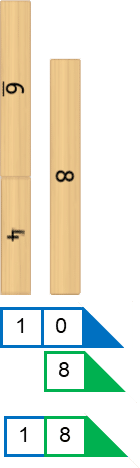##### Rule place value columns
• If placing the blocks correctly using place value is too difficult rule a line on the write and wipe sleeve to create place value columns.
• As the student increases in confidence erase the line.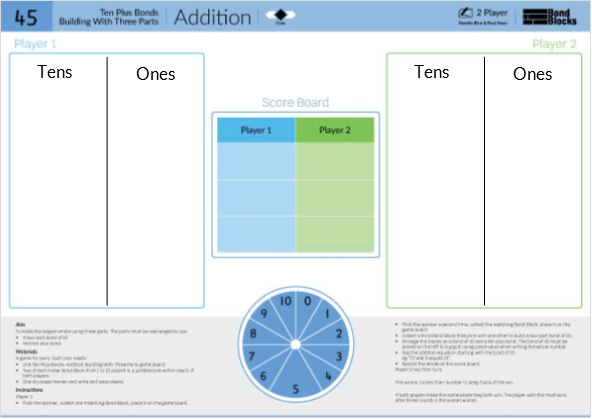### A little harder

##### Total after two rounds

Play for two rounds. After both rounds add both scores to make a cumulative total. The largest total is the winner.

For example, Player One scored 18 in the first round and 15 in the second to make a cumulative total of 33.Bond Blocks can be used to find the sum of each round. Organise Bond Blocks to support place value.

Provided below are two different examples of partitioning 18 + 15 to make addition easier. Both examples make a bond of 10.

In this example when calculating 15 + 18 the 8 is partitioned using a five plus bond to build ten.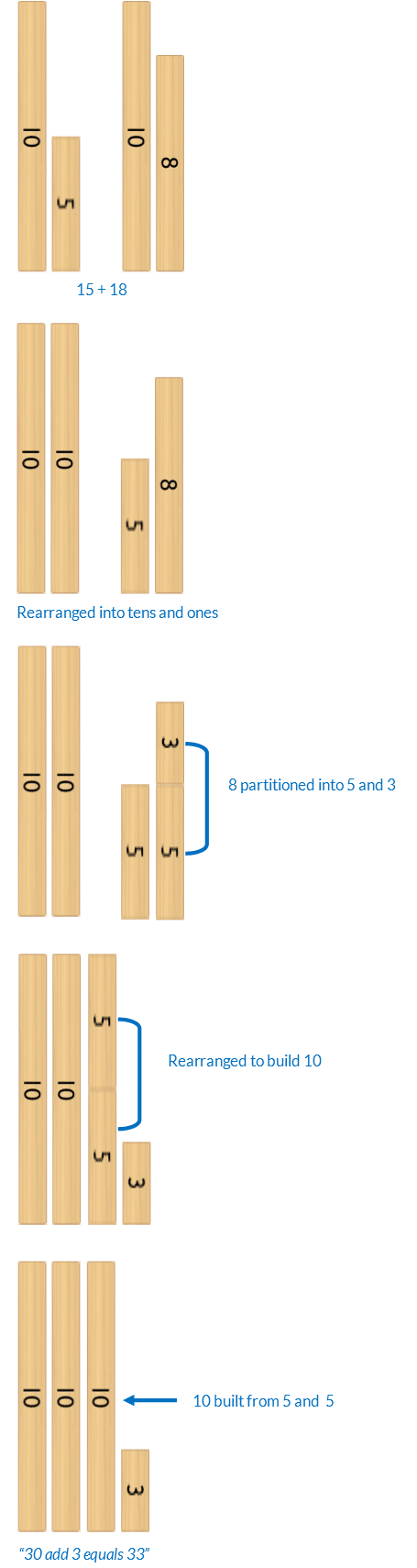In this example when calculating 15 + 18 the 5 is partitioned into 3 and 2 to build ten.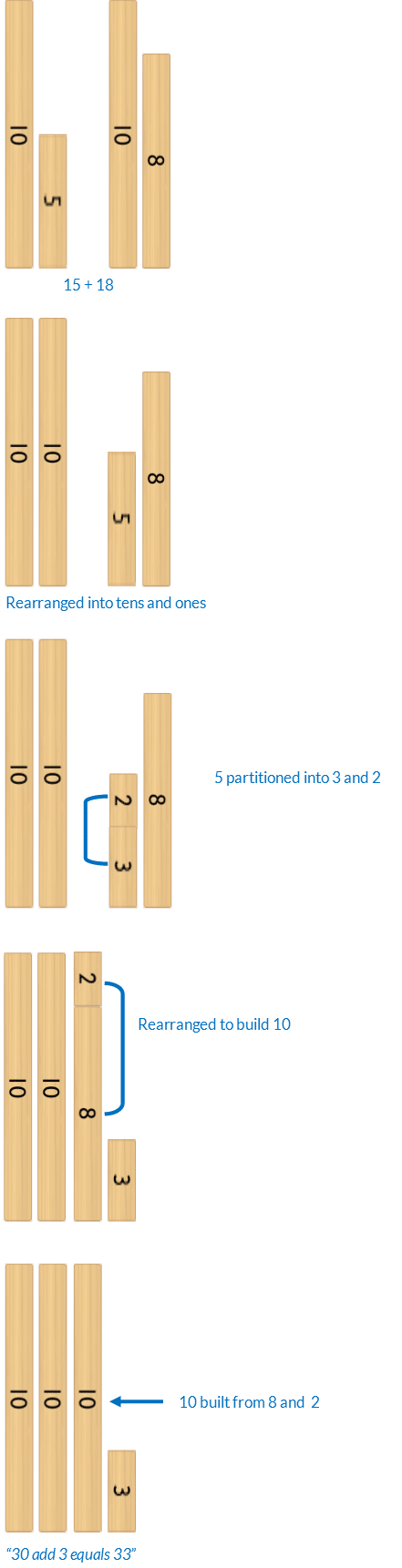### Progression

In the next activity students apply ten plus bonds to write addition and subtraction equations. Go to

##### Activity 46

Ten Plus Bonds: Equation, Building